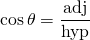# Unit 7 Right Angled Triangles

Right angled triangles have

• one angle that measures 90 degrees;
• a side called the hypotenuse which lies opposite the 90 degree angle;
• two smaller angles that add together to 90 degrees

In a problem involving only the sides of the right angled triangle, we use Pythagoras’ Theorem to solve it.In a problem involving an angle, we use trigonometry (the SOH CAH TOA formulae) to solve it.Explore here!